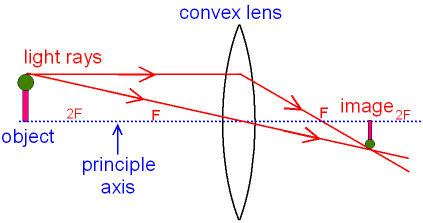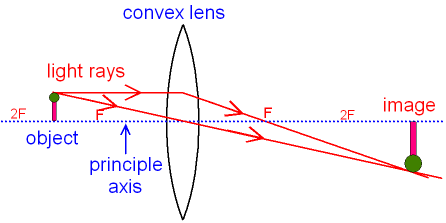gcsescience.com                                       27                                       gcsescience.com

Waves

Ray Diagrams for Images made by a Convex Lens.

The type of image made by a convex lens
depends on how far away the object is.

The first picture below shows how to draw a ray diagram
for an object that is further away from the lens than 2F.
Further down the page there is a picture showing
a ray diagram for an object that is between F and 2F.
an object that is nearer the lens than F.

F is at the focal point of the lens.
The distance from F to the centre of the lens is the focal length.
2F is twice the focal length.

R
ay diagram for an object that is
further away from the lens than 2F.

The bottom of the object is placed on the principle axis.
Two rays of light are drawn from the top of the object.
The first ray of light is parallel to the principle axis
and therefore passes through the focal point.
The second ray of light goes from the top of the object
and passes straight through the centre of the lens.The top of the image is formed where the two rays of light cross.
The bottom of the image is still on the principle axis.

You can see that the image is not the same as the object.
The image is smaller than the object.
The image is real meaning that the light rays really go there
(compare this with virtual).
The image is inverted (meaning it is upside down).

The next picture shows a ray diagram
for an object that is between F and 2F.As above, you can see that the image is
not the same as the object. The image is still real
and inverted but it is now bigger than the object.

gcsescience.com       Physics Quiz       Index       Lens Quiz       gcsescience.com